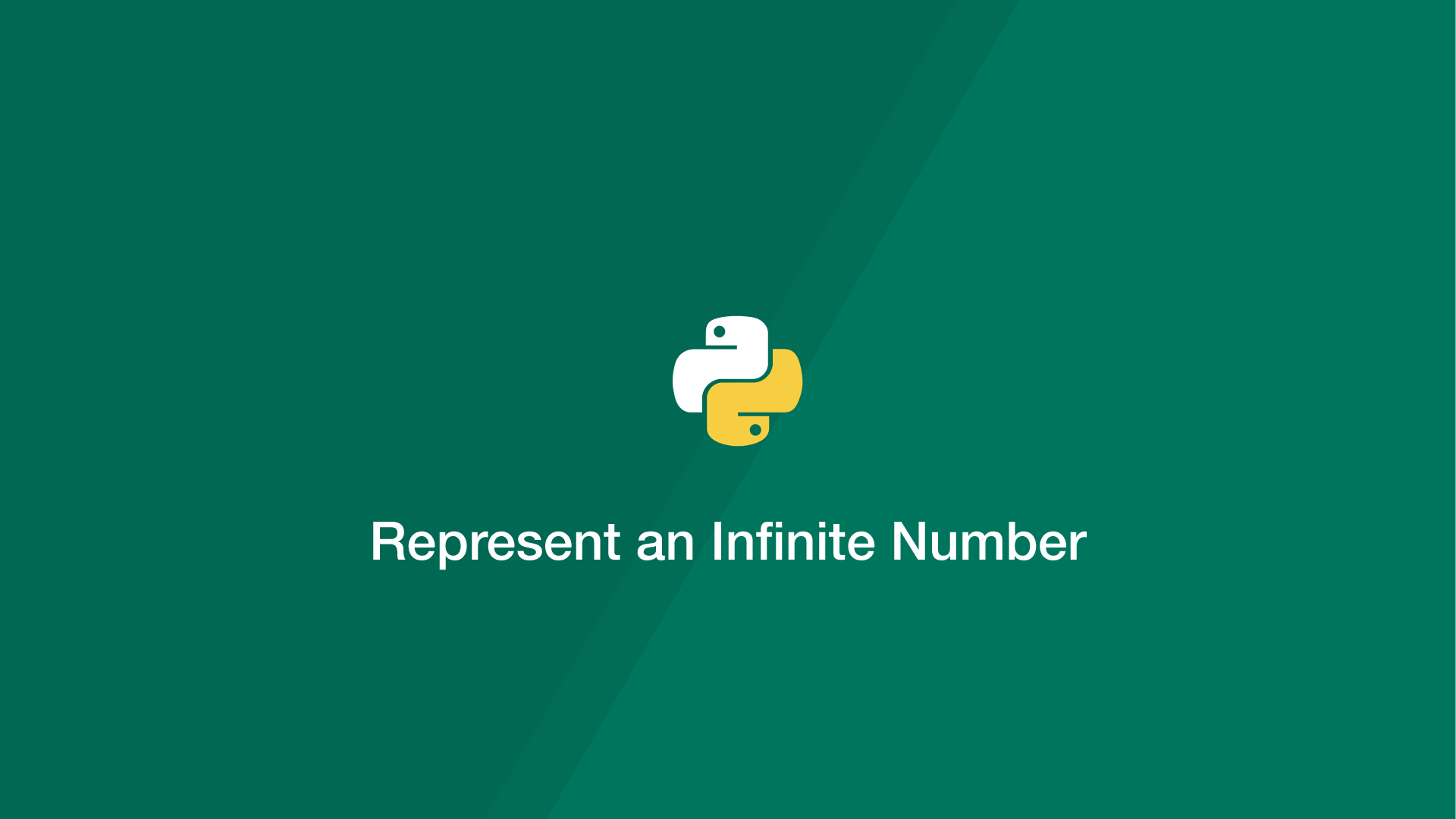# How to Represent an Infinite Number in PythonInfinity is an abstract number that can be negative or positive and represents both the small and largest possible number. In this tutorial, we will go through some of the different ways to use infinity in Python.

## Create Positive and Negative Infinity in Python Using float()

In Python, like many other popular programming languages, infinity is represented with the keyword `inf`. To create infinity in Python pass `'inf'` or '`-inf'` for negative as the first argument of the float() function like this:

``````#Positive infinity
infinity = float('inf')
print('Positive Infinity: ', infinity)

#Negative infinity
neg_infinity = float('-inf')
print('Negative Infinity: ', neg_infinity)
``````
``````Positive Infinity:  inf
Negative Infinity:  -inf
``````

## Use the math Package to Represent Positive and Negative Infinity in Python

Another way to create negative and positive infinity in Python is with the math package. Use the `math.inf` property for positive infinity and `-math.inf` for negative infinity.

``````#Positive infinity
infinity = float('inf')
print('Positive Infinity: ', infinity)

#Negative infinity
neg_infinity = float('-inf')
print('Negative Infinity: ', neg_infinity)
``````
``````Positive Infinity:  inf
Negative Infinity:  -inf
``````

## Check Number is Infinity in Python

To check a number is infinite in Python use the `isinf` function from the math package like this:

``````import math

#Positive infinity
infinity = math.inf
print('Positive Infinity: ', math.isinf(infinity))

#Negative infinity
neg_infinity = -math.inf
print('Negative Infinity: ', math.isinf(neg_infinity))
``````

## Represent Positive and Negative Infinity Using NumPy

To represent infinity using NumPy, use `np.inf` or `-np.inf`:

``````import numpy as np

#Positive infinity
infinity = np.inf
print('Positive Infinity: ', infinity)

#Negative infinity
neg_infinity = -np.inf
print('Negative Infinity: ', neg_infinity)
``````
``````Positive Infinity:  inf
Negative Infinity:  -inf
``````

## Represent Positive and Negative Infinity Using the Decimal Package

If you're using the Python decimal package you can represent negative and positive infinity with it like this:

``````import math
from decimal import Decimal

#Positive infinity
infinity = Decimal('Infinity')

#Negative infinity
neg_infinity = Decimal('-Infinity')

print('Positive Infinity: ', infinity)
print('Is Infinity: ', math.isinf(infinity))
print('Negative Infinity: ', neg_infinity)
print('Is Infinity: ', math.isinf(neg_infinity))
``````
``````Positive Infinity:  Infinity
Is Infinity:  True
Negative Infinity:  -Infinity
Is Infinity:  True
``````

#### Related Tutorials### How to Use While Loops in Python

September 03, 2020### How to Create Functions with Optional Arguments in Python

April 13, 2021### How to get the Absolute Value of a Number in Python

September 17, 2020### The Python Sleep Function

October 01, 2020### The Python zip() Function

October 04, 2020April 13, 2021